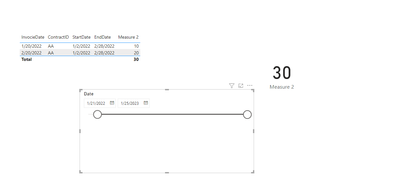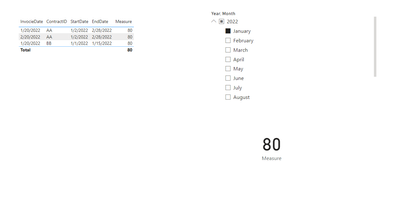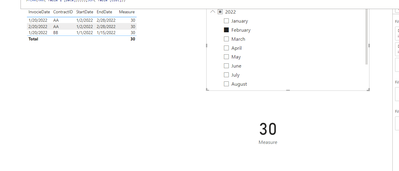cancel
Showing results for
Did you mean:Helper II

## Sum contract cost for contract that are/were active in a given period

I need to sum cost of contracts that were or are activ in a period given by a slicer using columns from date table.
this is a simplified version of tabel with contracts
All dates are dates.
Start and Enddate for a ContractID are always the same.
InvocieDate is key vs Date in date table

 InvocieDate ContractID StartDate EndDate Cost 2022-01-20 AA 2022-01-02 2022-02-30 10 2022-02-20 AA 2022-01-02 2022-02-30 20 2022-01-20 BB 2022-01-01 2022-01-15 50

If I set slicer to:
YearMonth = 2022 Jan
I want to get the sum 80

If I set slicer to:
YearMonth = 2022 feb
I want to get the sum 30

/Måns

1 ACCEPTED SOLUTIONCommunity Support

You can delete the relationship between two tables, then modified the measure

``````Measure 2 =
VAR mindate =
MIN ( 'Table 2'[Date] )
VAR maxdate =
MAX ( 'Table 2'[Date] )
VAR RESULT =
CALCULATE (
SUM ( 'Table'[Cost] ),
FILTER ('Table' , [EndDate] >= mindate && [StartDate] <= maxdate )
)
RETURN
RESULT``````Best Regards!

Yolo Zhu

If this post helps, then please consider Accept it as the solution to help the other members find it more quickly.

6 REPLIES 6Super User

Hi,

Hope this helps.Regards,
Ashish Mathur
http://www.ashishmathur.comCommunity Support

You can refer to the following example.

Create a new measure

``Measure = CALCULATE(SUM('Table'[Cost]),FILTER(ALL('Table'),EOMONTH([EndDate],0)>=EOMONTH(MAX('Table 2'[Date]),0)&&EOMONTH([StartDate],0)<=EOMONTH(MAX('Table 2'[Date]),0)))``Best Regards!

Yolo Zhu

If this post helps, then please consider Accept it as the solution to help the other members find it more quickly.Helper II

Awsome @v-xinruzhu-msft  ‌‌ this solves my issue as I stated it.

Unfotunetly there are some two circumstances to take in acount that I realise that I faild to include.

1, Messure needs to work for periods ranging from a single date to period ranging over all dates.
2, Messure should show blank if visulaised in a tabel for rows were contract is inactive also I need it to not show same values an all rows. like below with date slicer: 2022-01-25 - 2022-01-31

 invocieDate ContractID StartDate EndDate Measure 2022-01-20 AA 2022-01-02 2022-02-28 10 2022-02-20 AA 2022-01-02 2022-02-28 20 2022-01-20 BB 2022-01-01 2022-01-15 total 30

I´ve solved issue 1 by modifying you´re awsome measure like this:

Measure 2 =
VAR mindate =
MIN ( 'Table 2'[Date] )
VAR maxdate =
MAX ( 'Table 2'[Date] )
VAR RESULT =
CALCULATE (
SUM ( 'Table'[Cost] ),
FILTER ( ALL ( 'Table' ), [EndDate] >= mindate && [StartDate] <= maxdate )
)
RETURN
RESULT

Issue 2 is an other matter I´ve tride resolving it using SUMX with diffrent filtering and stuff but I´m stuck ‌‌

Would you mind taking an other look?Community Support

You can delete the relationship between two tables, then modified the measure

``````Measure 2 =
VAR mindate =
MIN ( 'Table 2'[Date] )
VAR maxdate =
MAX ( 'Table 2'[Date] )
VAR RESULT =
CALCULATE (
SUM ( 'Table'[Cost] ),
FILTER ('Table' , [EndDate] >= mindate && [StartDate] <= maxdate )
)
RETURN
RESULT``````Best Regards!

Yolo Zhu

If this post helps, then please consider Accept it as the solution to help the other members find it more quickly.Helper II

Turned out I needed that relationship for other stuff.
I changed my measure by wraping code in an adiddional calculate and adding crossfilter to it like this.

Measure 3 =
VAR mindate =
MIN ( 'Table 2'[Date] )
VAR maxdate =
MAX ( 'Table 2'[Date] )
VAR RESULT =
CALCULATE(
CALCULATE (
SUM ( 'Table'[Cost] ),
FILTER ( 'Table' , [EndDate] >= mindate && [StartDate] <= maxdate )
),
CROSSFILTER('Table 2'[Date],'Table'[InvocieDate],None))
RETURN
RESULT
Thanks for the help everyone.Super User